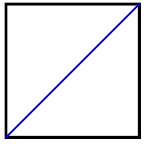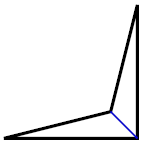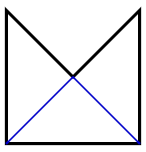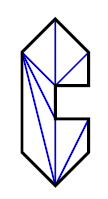### Problem Statement

A simple polygon is one where none of the sides of the polygon touch each other, except when adjacent edges touch at their endpoints. Any simple polygon can be triangulated into a number of triangles by drawning N-3 line segments between pairs of the polygon's vertices, where N is the total number of vertices. The N-3 lines must not touch each other, or any of the edges of the polygon, except where they touch at their endpoints.

Your task is, given a simple polygon, find a triangulation for this polygon. The polygon will be given as two int[]s, x and y, where there is an edge from x[i],y[i] to x[(i+1)%N],y[(i+1)%N]. You should return this triangulation as a String[], where each element is of the form "P1 P2", where P1 and P2 are the 0-based indices of the vertices in the input, and P1 < P2. Your return should be sorted first by P1, and ties should be broken by P2. If there are multiple possible triangulations, find the first element of the return for which the two differ. Choose the triangulation that has the smaller P1 in this element. If there is a tie, choose the one with the smaller P2. For example, choose {"0 2"} over {"1 3"} and {"0 2","0 3","2 4"} over {"0 2","0 4","1 3"}.

### Definition

 Class: Triangulation Method: triangulate Parameters: int[], int[] Returns: String[] Method signature: String[] triangulate(int[] x, int[] y) (be sure your method is public)

### Constraints

-x and y will each contain between 4 and 50 elements, inclusive.
-x and y will contain the same number of elements.
-Each element of x and y will be between -1000 and 1000, inclusive.
-The input will represent a simple polygon, where each edge has length greater than 0, and no two adjacent edges are parallel.

### Examples

0)

 `{0,10,10,0}` `{0,0,10,10}`
`Returns: { "0 2" }`
 This input represents a square from (0,0) to (10,10), which can be triangulated by drawing a line from (0,0) to (10,10).1)

 `{0,10,10,8}` `{0,0,10,2}`
`Returns: { "1 3" }`2)

 `{0,5,10,10,0}` `{10,5,10,0,0}`
`Returns: { "1 3",  "1 4" }`3)

 `{0,1,1,0,0,1,1,0,-1,-1}` `{0,1,2,2,3,3,4,5,4,1}`
`Returns: { "0 2",  "0 3",  "0 8",  "3 8",  "4 6",  "4 7",  "4 8" }`4)

 `{0,1,1,0,1,1,0,-1,-1}` `{0,1,2,2,3,4,5,4,1}`
`Returns: { "0 2",  "0 3",  "0 7",  "3 5",  "3 6",  "3 7" }`

#### Problem url:

http://www.topcoder.com/stat?c=problem_statement&pm=3485

#### Problem stats url:

http://www.topcoder.com/tc?module=ProblemDetail&rd=5871&pm=3485

lars2520

#### Testers:

PabloGilberto , brett1479

Geometry